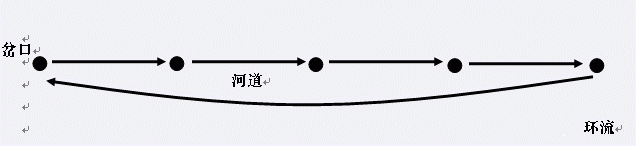# description

vani和cl2在一片树林里捉迷藏……由于人数众多的原因，Y族的祭祀活动会在多个岔口上同时举行。出于对龙王的尊重，这些祭祀地点的选择必

# analysis

• 考场想了个$naive$的伪正解

• $O(n^2)$处理出两两点是否可达，然后相当于求一个最大完全图，其中两两点互不可达

• 不就求一个最大团，但是$T90$，怎么搞都过不了

• 正解先用$floyd$搞一个什么傻逼传递闭包（对就是预处理两两点是否可达）

• 因为最小路径覆盖数$=n-$二分图最大匹配数，于是拆点然后跑一个匈牙利就行

• 大概就这样吧

# code

#pragma GCC optimize("O3")
#pragma G++ optimize("O3")
#include<stdio.h>
#include<string.h>
#include<algorithm>
#define MAXN 205
#define ll long long
#define reg register ll
#define fo(i,a,b) for (reg i=a;i<=b;++i)
#define fd(i,a,b) for (reg i=a;i>=b;--i)

using namespace std;

bool bz[MAXN][MAXN];
bool flag[MAXN];
ll a[MAXN][MAXN];
ll f[MAXN];
ll n,m,ans;

{
ll x=0,f=1;char ch=getchar();
while (ch<'0' || '9'<ch){if (ch=='-')f=-1;ch=getchar();}
while ('0'<=ch && ch<='9')x=x*10+ch-'0',ch=getchar();
return x*f;
}
inline bool find(ll x)
{
fo(i,1,a[x])if (flag[a[x][i]])
{
flag[a[x][i]]=0;
if (!f[a[x][i]] || find(f[a[x][i]])){f[a[x][i]]=x;return 1;}
}
return 0;
}
int main()
{
freopen("T2.in","r",stdin);